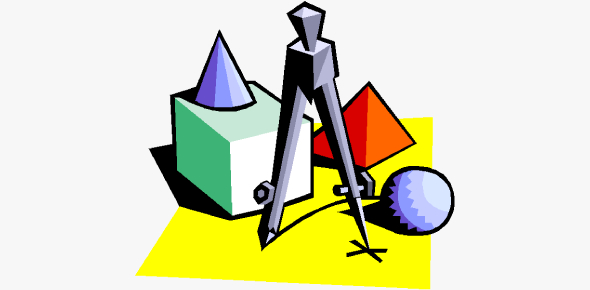# Geometry Honors Quiz: Test!

17 Questions | Attempts: 146Settings.

• 1.
Conjecture: If an obtuse angle is bisected, then the two newly formed congruent angles are______.
• A.

Right angles

• B.

Congruent angles

• C.

Acute angles

• D.

Obtuse angles

• E.

None of the above

• 2.
If two angles form a linear pair, then ______________________________________
• 3.
If two angles are vertical angles, then their measures are _____________.
• A.

Congruent

• B.

Equal

• C.

90 degrees

• D.

Large

• E.

Both a and b

• 4.
What is the definition of a converse?
• 5.
If two parallel lines are cut by a _________, then corresponding angles are congruent.
• A.

Transvex

• B.

Line

• C.

Ray

• D.

Alternate angle

• E.

None of the above

• 6.
If a transversal cuts two parallel lines, then alternate interior angles are congruent, and alternate _________ angles are congruent.
• A.

Exterior

• B.

Interior

• C.

Opposite

• D.

Other

• E.

None of the above

• 7.
Is the converse of the Parallel Lines Conjecture (If a transversal cuts two parallel lines, then corresponding angles are congruent, alternate interior angles are congruent, and alternate exterior angles are congruent) true?
• A.

Yes

• B.

No

• 8.
When you duplicate an angle, do you ever change the compass setting?
• A.

Yes

• B.

No

• C.

Depends

• 9.
When constructing any parts of geometry, what are the only two tools you can use?
• A.

Compass

• B.

Protractor

• C.

Ruler

• D.

Straightedge

• E.

Both a and d

• 10.
A median is ___________________________________
• A.

The line connecting the vertex of a triangle to the midpoint of its opposite side

• B.

The segment connecting the vertex of a triangle to a point of its opposite side

• C.

The ray connecting the midpoint of a segment of a triangle to a midpoint of its opposite side

• D.

The segment connecting the vertex of a triangle to the midpoint of its opposite side

• E.

None of the above

• 11.
A midsegment is........
• 12.
What does equidistant mean?
• 13.
If a point is on the ________________ bisector of a ____________, then it is equidistant from the endpoints.
• A.

Perpendicular, ray

• B.

Parallel, segment

• C.

Perpendicular, segment

• D.

• E.

None of the above

• 14.
The _____________ distance from a point to a line is measured along the perpendicular from the point to the line.
• A.

Longest

• B.

Equal

• C.

Shortest

• D.

Easiest

• E.

None of the above

• 15.
What is the definition of an altitude?
• 16.
What two ways can you make constructions?
• A.

Patty paper

• B.

Compass and straightedge

• C.

Ruler and protractor

• D.

Both a and b

• E.

Both a and c

• 17.
Is the converse of the Vertical Angles Conjecture true or false?
• A.

True

• B.

False

## Related TopicsBack to top
×

Wait!
Here's an interesting quiz for you.